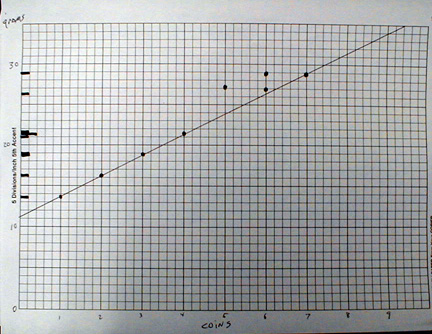Filmcan Periodic Table

Investigate the weights of film cans and create a periodic table.

Material

• 6 identical film cans per experiment
• 35 identical pennies, make sure you use only pennies minted after 1983, the composition of pennies changed that year. These pennies have a mass of 2.5 grams.
• optional, use washers, find washers with diameters that just barely fit inside the film cans, I use 7/16 inch washers, that's the inside diameter.
• a digital scale capable of reading to 0.1 gram
• graph paper

Assembly

Check the masses of the film cans, In my sample of film cans, I kept film cans that had a mass between 5.9 and 6.0 grams and excluded all others. You must do this. (Film can masses vary treemendously)

Put the following numbers of washers in the film cans: 1,4, 7,9,11,12

Label the cans with the element symbols: U,D,T,Q,P,H.

If you have more than one group of 6 film cans continue with 14,16,19,20.

Label these cans S,O,N,De.

To Do and Notice

Work out the nuclear composition of these elements.

Each group of investigators gets a set of 6 cans.
Tell the group the mass of the empty cans, for my set this was 6.0 g +- 0.1 g.
Each can contains one "nole" of atoms of an element, 10^10 atoms. Not a mole, a nole.

Use the digital scale to measure the masses of the cans.

Arrange the cans in order by mass.

Make a table of the masses of a nole of the element in each can (subtract the mass of the can.)
Make a table of the differences in the masses of a nole of each element compared to that of the nearest heavier neighbor.

Plot the masses on a number line.

Do you see a pattern to the masses?

( Optional, Combine data from different teams onto one number line.)

Convert the number line plot into a y versus x graph.
Where the y axis is the mass of a nole of each element.
The x axis is a number associated with each can that produces a straight line graph.

The slope of this line has units of grams per penny, it is equal to the mass of one penny assuming that at least two cans differed in mass by only 1 penny.

Based on your measurements, and without removing any washers from their cans!

What is the mass of a penny?

Can you calculate the mass of the film can from the data? What must you assume to calculate this mass?

After you have determined the masses of the film can and washer from the data you can then remove a washer from a film can and check the answer.

You can also do the number line plot and graph using the differences in masses between cans and their closest mass neighbors.Here is an example of the data analysis (On this graph the mass of the film can was not subtracted.)

Plot the masses in grams of the film cans with coins or washers on the y axis.

Notice the spacing of the masses.

Assign probable numbers of coins in each can, note that two initial guesses for the numbers of coins do not lie on a line, changing the estimated number of coins in the cans makes the masses lie on a line.

The slope of the line is in grams per coin and is the mass of a coin.

What's Going On?

In the real periodic table, the elements were initially arranged in order of atomic mass.

A look at the differences in the atomic masses between one element and the next revealed patterns of mass increase that indicated that the mass of each element was going up in integer multiples of a basic mass. The basic mass unit was the mass of a proton or neutron. This pattern is particularly clear if isotopically pure specimens of elements are used.

The most common isotope of hydrogen has 1 proton, and a mass of about 1 amu.
An amu is an atomic mass unit.

Helium's common isotope has 2 protons and 2 neutrons and has mass about 4 amu.

Lithium has 3 protons and 4 neutrons, mass 7 amu

Many elements have more than one stable isotope with different masses, however for the lighter elements one isotope is usually much more common and so the resulting mass is near an integer number of amu.

If you use the numbers of coins that I suggest you will recreate the pattern of masses found in most common isotopes of the first elements of the periodic table.

References

The pictorial Periodic table : http://140.198.18.108/periodic/about.html
A table of the isotopes of the elements.

The Periodic Kingdom, P.W. Atkins, Basic Books, 1995. An excellent Introduction to the Periodic Table for teachers and students.

 Scientific Explorations with Paul Doherty © 2000 17 November 2000

Going Further

Making the periodic table.

I've created my own elements (Inspired by a two dimensional universe.)

Here is a table of element names and isotopic masses.

Element Names

 Name atomic symbol Washer Number Unium U 1 Duium D 4 Tertium T 7 Quartium Q 9 Pentium P 11 Hexium H 12 Septium S 14 Ottium O 16 Nonium N 19 Decium De 20

To make a real periodic table you need to know how these elements react:

The following compounds are formed:

D and O make no compounds.

• US, TS, NS
• U2H, T2H, N2H
• QS2 , DeS2
• PU3, PT3, PN3

You can use these compounds to figure out the valence of the atoms in my world and start to fill in a periodic table.

Can you predict some other possible compounds?